Stella Dimoko Korkus.com: BBNaija Season 8 All Stars - Pere Declares Love For Alex, Frodd Breaks Down + Other Gists

## Wednesday, August 09, 2023

### BBNaija Season 8 All Stars - Pere Declares Love For Alex, Frodd Breaks Down + Other Gists

This Big brother house is what i have tagged as DOUBLE WAHALA......

<<<<<<<<<<<<<<<<<<<<<<<<<<<<<<<<<<<<<<<<<<<<<<<<<<<<<<<<<<<<<<<<<<<<<<<<<<<<<<<<<<<<<<<<<<<<<<<<<<<<<<<<<<<<<<<<<<<<<<<<<<<<<<<<<<<<<<<<<<<<<<<<<<<<<<<<<<<<<<<<<<<<<<<<<<<<<<<<<<<<<<<<<<<<<<<<<<<<<<<<<<<<<<<<<<<<<<<<<<<<<<<<<<<<<<<<<<<<<<<<<<<<<<<<<<<<<<<<<<<<<<<<<<<<<<<<<<<<<<<<<<<<<<<<<<<<<<<<<<<<<<<<<<<<<<<<<<<<<<<<<<<<<<<<<<<<<<<<<<<<<<<<<<<<<<<<<<<<<<<<<<<<<<<<<<<<<<<<<<<<<<<<<<<<<<<<<<<<<<<<<<<<<<<<<<<<<<<<<<<<<<<<<<<<<<<<<<<<<<<<<<<<<<<<<<<<<<<<<<<<<<<<<<<<<<<<<<<<<<<<<<<<<<<<<<<<<<<<<<<<<<<<<<<<<<<<<<<<<<<<<<<<<<<<<<<<<<<<<<<<<<<<<<<<<<<<<<<<<<<<<<<<<<<<<<<<<<<<<<<<<<<<<<<<<<<<<<<<<<<<<<<<<<<<<<<<<<<<<<<<<<<<<<<<<<<<<<<<<<<<<<<<<<<<<<<<<<<<<<<<<<<<<<<<<<<<<<<<<<<<<<<<<<<<<<<<<<<<<<<<<<<<<<<<<<<<<<<<<<<<<<<<<<<<<<<<<<<<<<<<<<<<<<<<<<<<<<<<<<<<<<<<<<<<<<<<<<<<<<<<<<<<<<<<<<<<<<<<<<<<<<<<<<<<<<<<<<<<<<<<<<<<<<<<<<<<<<<<<<<<<<<<<<<<<<<<<<<<<<<<<<<<<<<<<<<<<<<<<<<<<<<<<<<<<<<<<<<<<<<<<<<<<<<<<<<<<<<<<<<<<<<<<<<<<<<<<<<<<<<<<<<<<<<<<<<<<<<<<<<<<<<<<<<<<<<<<<<<<<<<<<<<<<<<<<<<<<<<<<<<<<<<<<<<<<<<<<<<<<<<<<<<<<<<<<<<<<<<<<<<<<<<<<<<<<<<<<<<<<<<<<<<<<<<<<<<<<<<<<<<<<<<<<<<<<<<<<<<<<<<<<<<<<<<<<<<<<<<<<<<<<<<<<<<<<<<<<<<<<<<<<<<<<<<<<<<<<<<<<<<<<<<<<<<<<<<<<<<<<<<<<<<<<<<<<<<<<<<<<<<<<<<<<<<<<<<<<<<<<<<<<<<<<<<<<<<<<<<<<<<<<<<<<<<<<<<<<<<<<<<<<<<<<<<<<<<<<<<<<<<<<<<<<<<<<<<<<<<<<<<<<<<<<<<<<<<<<<<<<<<<<<<<<<<<<<<<<<<<<<<<<<<<<<<<<<<<<<<<<<<<<<<<<<<<<<<<<<<<<<<<<<<<<<<<<<<<<<<<<<<<<<<<<<<<<<<<<<<<<<<<<<<<<<<<<<<<<<<<<<<<<<<<<<<<<<<<<<<<<<<<<<<<<<<<<<<<<<<<<<<<<<<<<<<<<<<<<<<<<<<<<<<<<<<<<<<<<<<<<<<<<<<<<<<<<<<<<<<<<<<<<<<<<<<<<<<<<<<<<<<<<<<<<<<<<<<<<<<<<<<<<<<<<<<<<<<<<<<<<<<<<<<<<<<<<<<<<<<<<<<<<<<<<<<<<<<<<<<<<<<<<<<<<<<<<<<<<<<<<<<<<<<<<<<<<<<<<<<<<<<<<<<<<<<<<<<<<<<<<<<<<<<<<<<<<<<<<<<<<<<<<<<<<<<<<<<<<HOUSEMATE PERE CONFESSES HE IS IN LOVE WITH CO HOUSEMATE ALEX

Pere had a conversation with Uriel, Venita and Ike,and revealed his feelings for Alex and said he can forfeit the N120 million prize money for her sake.
He said, "There is only one girl here I’m in love with. I’m ready to drop the N120 million for this girl.”
Uriel responded, “I will be happy to attend your wedding if it ever gets to that point.”

#########

HOUSEMATE FRODD SENDS EMOTIONAL MESSAGE TO PREGNANT WIFE

In his diary session, Frodd sent an emotional message to his heavily pregnant wife whose EDD is close.

He said, "I know what this week means to you, I know what it means to us. I pray every day, and I’m still praying for you till now. I wish you the best of luck, my love; I wish you the best of luck, my baby. I wish you everything good. I know I won’t be there, but I want you to know that I love you, and I want you to know that you’re good and you’ll be fine.”

############

HOUSEMATE DOYIN SAYS SHE IS ATTRACTED TO KIDDWAYA

Doyin who chatted with CeeCee revealed her attraction to Kiddwaya.
She recounted how he checked up on her after her altercation with Cross. She said that she isn't particularly fond of his character, but she can set his character aside and "f*ck his brains out."

CeeCee smiled and said that Kidd is a cool person.

###################

Adekunlke who had a convo with Seyi and Venitasaid he doubts Whitemoney is who he says he is.
He recounted a conversation he had with Whitemoney, where he (Whitemoney) told him he has a car business. Out of curiosity, he (Adekunle) asked him the price of a particular car and Whitemoney had no idea.

He said that the incident made him doubt his stories of the luxurious life he lives outside.

###################

HOUSEMATE WHITEMONEY'S INSTAGRAM ACCOUNT HAS BEEN HACKED

Housemate,Whitemoney’s verified Instagram account has been hacked.
The account with 1.9 million followers was hacked around 12 am on Tuesday August 8, 2023.
The account was put on ‘private’.by the person who took it.

From dailypost

1.Adekunle never keeps quiet. Leave WM face your game you are ALL liars in that house no saint. 🤔🤔🤔

1.He expect WM to stay in the house and know the price of car outside. Like prices of things must always remain the same.
I thought they said he’s the only male housemate who does not gossip ikwakwakwakwaeeee

Fan Emmanuel

2.I don't want Seyi to leave so early, I will be broken if he does. I so love that guy, I don't know why some hate him. Kindly vote my Sucre Papito

2.Hahaha Doyin! Kiddwaya na cruise o. Pere X Alex wow Okay now

I feel for Frodd oh, this is his first child oh I wish there was a way for him to there with his wife codely. Congratulations!

Whitemoney account hacked. Wrong timing mehn!

3.Frodd, your wife will be fine. I wish her a successful delivery.

4.Happy Women Crush Wednesday to all the strong women out there.
Side eyes to men that always compete with women here.

5.Happy Women Crush Wednesday to all the strong women out there.
Side eyes to men that always compete with women here.

6.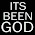Pere, stay one place and leave our Alex alone, jare. You know it's all fake. Abeggi!

Kiddwaya's diary session was just so funny. That guy na real case! I don laugh taya. Terry Waya's son dey okay politics but got played. 😂

Frodd, your wife and baby will be fine by God's grace.

Doyin, so Chizzy the rider is no longer in the picture abi na game too? Issokai!

1.She said she liked Chizzy but can't date him because he's not her type,that hes loud and she doesn't like loud guys but they're cool friends.

2.This is different from what she said in a video that I saw. In fact, she was angry that people were tweeting that Chizzy is not up to her in level and class.

3.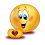I laughed all through kids dairy section

Pere is fake you liked Alex promised to pardon her but went ahead to pardon cross and told cross to lie to her that you pardoned her

7.FROOD. All will be well with your wife and baby.
This people are taking this game to another level. Why hack whitemoney s account at this critical time. Anyway God no be man.

1.Hope his former handler is innocent. I don’t know why he decided to accuse the guy of eating his money at this time

Fan Emmanuel

8.Hmmmm Pere! Are you sure this is not a strategy? I know he will always have a thing for Maria sha though she is expecting now.

1.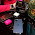Definitely a strategy cos how?? He didn't see beautiful Uriel to fall in love with or what

2.I think Pere likes them fair, just my opinion

9.Alex should be careful with Pere, the conversation he had with Cross says otherwise. Adekunle should leave Whitemoney alone, because he did not readily tell him the prize of a vehicle does not mean he is not a dealer

10.Doyin is a wild one. Leos don't play 😊

11.Good luck to Pelex
Please let Pere and Alex be in the house long,I want to see 🤣🤣
something

Safe delivery to Frood's wife..

Doyin hmmm!!!
Be focus

Too much gossip in the house 🤣🤣🤣

Whitemoney's account hacked chai!!!

12.Alex please don't fall for this,it's a strategy!
Doyin is a confused babe.

13.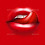I don't trust my general Perez feeling towards my girl Alex ,but if it genuine I will gladly ship them 😁

Doyin Doyin Doyin!!! how many times did I call you? In whatever you do,avoid Kidd..he's the original cruise master,him go kid you along and bounce

Frood I wish your wife safe delivery

Deks the badt guy,biko leave white money alone..if he chooses to lie,what do you care? Leave him to his conscience! Focus focus focus on your own game and strategy

1.exactly, i dont trust pere!

2.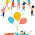I don't trust Pere at all.

14.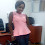Pere, abeg face ya front and leave our Alex for now. You can go for her outside biggies house biko.
Doyin crushing on Kid, okay na. More power to ya muscle.
Congratulations Frodd. Your wify will deliver safely and be fine kkkkkk

15.PERE IS A BEAST OF THIS GAME AND A VERY VINDICTIVE PERSON!!! YOU SUDDENLY HAVE FEELINGS FOR ALEX BECAUSE YOU HAVE FALLEN OUT WITH CEEC??? LMAO IT'S GIVING THE ENEMY OF MY ENEMY IS MY FRIEND!!! YOU RE TOO OLD FOR THIS SLITHERY BEHAVIOUR!!! YOUR DESPERATION FOR THE GAME IS GIVING YOU OUT!!!

1.Actually the fallout with Ceece was because Pere didn't support her when Ceece and Alex had altercation. This much was revealed during life eviction show where Pere said Neo told him and Ceece never challenged nor deny it.

16.Pere is lying 🤥

17.Pere please leave our Alex alone, Doyin wake up!
Frood your wife will be good.

18.I don't have any trust in Pere, he's a gamer. Safe delivery to your wife Frodd.

19.20.They all are gamers in that house and have all admitted to knowing that. As for Adekunle..he should focus on his own game and allow others do their own thing. Why carry searchlight about looking for people's strategy?
Nay the best gamer win

21.Pere leave my Alex to focus and play that game with another. We are enjoying this season.

Disclaimer: Comments And Opinions On Any Part Of This Website Are Opinions Of The Blog Commenters Or Anonymous Persons And They Do Not Represent The Opinion Of StellaDimokoKorkus.com

Pictures and culled stories posted on this site are given credit and if a story is yours but credited to the wrong source,Please contact Stelladimokokorkus.com and corrections will be made..